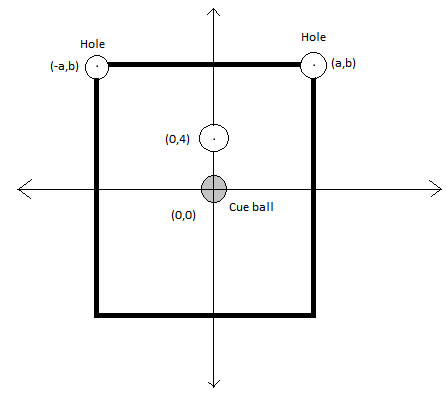# Let's play pool

Geometry Level 5A pool table is set up on a co-ordinate plane as shown in the figure. A cue ball of radius $r$ is placed with it's centre at the origin.Also the there are only two holes in the table each of radius $r$ with their centres at $(a,b)$ and $(-a,b)$ respectively.

The ball which is to be shot into the hole is of radius $r$ and is placed with it's centre at $(0,y)$.

If the possible values of $y$ for which it is possible to shoot the ball into the hole is $(c,d)$ that is $y\in(c,d)$. Then find ${c}^{2}+{d}^{2}$.

Details and Assumptions

1)$a=\frac { 5\sqrt { 3 } +\sqrt { 15 } }{ 2 },b=\frac { 9+\sqrt { 5 } }{ 2 },r= \frac{1}{2}$,Mass of both the balls is same.There is no friction on the table

2)The collision of the cue ball with the given ball is elastic.

3)The ball has to be directly shot into the hole.No bouncing with the sides of the pool table is allowed.

4)c,d are denoting the end-points of the range y is belonging to.

Sorry by mistake in the figure the co-ordinates of the ball is shown to be (0,4). It is actually (0,y)

×